# 案例加源码：万字长文带你彻底搞懂 MySQL 的索引优化# 建表

CREATE TABLE IF NOT EXISTS article(    id INT(10) UNSIGNED NOT NULL PRIMARY KEY AUTO_INCREMENT,    author_id INT(10) UNSIGNED NOT NULL,    category_id INT(10) UNSIGNED NOT NULL,    views INT(10) UNSIGNED NOT NULL,    comments INT(10) UNSIGNED NOT NULL,    title VARCHAR(255) NOT NULL,    content TEXT NOT NULL);INSERT INTO article(author_id,category_id,views,comments,title,content)VALUES(1,1,1,1,'1','1'),(2,2,2,2,'2','2'),(1,1,3,3,'3','3');View Code

# 查询案例

• 查询 category_id 为 1 且 comments 大于 1 的情况下，views 最多的 article_id 。

• 查询语句：SELECT id, author_id FROM article WHERE category_id = 1 AND comments > 1 ORDER BY views DESC LIMIT 1;

• 分析语句：EXPLAIN SELECT id, author_id FROM article WHERE category_id = 1 AND comments > 1 ORDER BY views DESC LIMIT 1;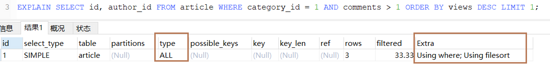• 分析：可看到上述 type 为 ALL ，证明未命中索引，并且 Using filesort 使用了文件排序 。故而可进行优化到使用索引。

# 优化一：

• 创建索引 ：CREATE INDEX idx_article_ccv ON article(category_id,comments,views);

• 查看当前索引：SHOW INDEX FROM article;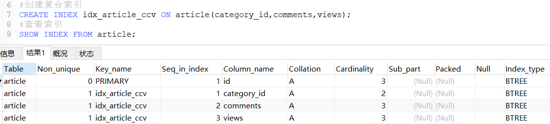• 查看执行计划 ：EXPLAIN SELECT id, author_id FROM article WHERE category_id = 1 AND comments > 1 ORDER BY views DESC LIMIT 1;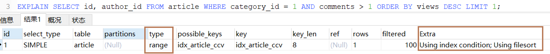• 分析：( 删除索引：DROP INDEX idx_article_ccv ON article;)因为按照 B+Tree 索引的工作原理，先排序 category_id，如果遇到相同的 category_id 则再排序 comments，如果遇到相同的 comments 则再排序 views。最左前缀匹配原则， 当 comments 字段在联合索引里处于中间位置时，因为 comments>1 条件是一个范围值（所谓 range），MySQL 无法利用索引再对后面的 views 部分进行检索，即 range 类型查询字段后面的索引无效。如果将条件 comments > 1 改成 comments = 1，则当前索引为类型为 ref，并且不存在 filesort。但是不满足题意

# 优化二：

• 创建索引：create index idx_article_ccv on article(category_id, views);

• 查看当前索引：SHOW INDEX FROM article;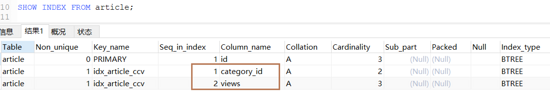• 再次 查看执行计划 ：EXPLAIN SELECT id, author_id FROM article WHERE category_id = 1 AND comments > 1 ORDER BY views DESC LIMIT 1;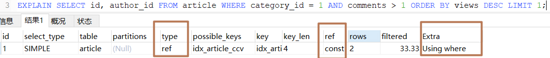• 可看到此时满足条件，为方便后续测试此时依然删除当前索引：DROP INDEX idx_article_ccv ON article;

# 建表

CREATE TABLE IF NOT EXISTS class(    id INT(10) UNSIGNED NOT NULL AUTO_INCREMENT,    card INT(10) UNSIGNED NOT NULL,    PRIMARY KEY(id));CREATE TABLE IF NOT EXISTS book(    bookid INT(10) UNSIGNED NOT NULL AUTO_INCREMENT,    card INT(10) UNSIGNED NOT NULL,    PRIMARY KEY(bookid));INSERT INTO class(card) VALUES(FLOOR(1+(RAND()*20)));INSERT INTO class(card) VALUES(FLOOR(1+(RAND()*20)));INSERT INTO class(card) VALUES(FLOOR(1+(RAND()*20)));INSERT INTO class(card) VALUES(FLOOR(1+(RAND()*20)));INSERT INTO class(card) VALUES(FLOOR(1+(RAND()*20)));INSERT INTO class(card) VALUES(FLOOR(1+(RAND()*20)));INSERT INTO class(card) VALUES(FLOOR(1+(RAND()*20)));INSERT INTO class(card) VALUES(FLOOR(1+(RAND()*20)));INSERT INTO class(card) VALUES(FLOOR(1+(RAND()*20)));INSERT INTO class(card) VALUES(FLOOR(1+(RAND()*20)));INSERT INTO class(card) VALUES(FLOOR(1+(RAND()*20)));INSERT INTO class(card) VALUES(FLOOR(1+(RAND()*20)));INSERT INTO class(card) VALUES(FLOOR(1+(RAND()*20)));INSERT INTO class(card) VALUES(FLOOR(1+(RAND()*20)));INSERT INTO class(card) VALUES(FLOOR(1+(RAND()*20)));INSERT INTO class(card) VALUES(FLOOR(1+(RAND()*20)));INSERT INTO class(card) VALUES(FLOOR(1+(RAND()*20)));INSERT INTO class(card) VALUES(FLOOR(1+(RAND()*20)));INSERT INTO class(card) VALUES(FLOOR(1+(RAND()*20)));INSERT INTO class(card) VALUES(FLOOR(1+(RAND()*20)));INSERT INTO class(card) VALUES(FLOOR(1+(RAND()*20)));INSERT INTO book(card) VALUES(FLOOR(1+(RAND()*20)));INSERT INTO book(card) VALUES(FLOOR(1+(RAND()*20)));INSERT INTO book(card) VALUES(FLOOR(1+(RAND()*20)));INSERT INTO book(card) VALUES(FLOOR(1+(RAND()*20)));INSERT INTO book(card) VALUES(FLOOR(1+(RAND()*20)));INSERT INTO book(card) VALUES(FLOOR(1+(RAND()*20)));INSERT INTO book(card) VALUES(FLOOR(1+(RAND()*20)));INSERT INTO book(card) VALUES(FLOOR(1+(RAND()*20)));INSERT INTO book(card) VALUES(FLOOR(1+(RAND()*20)));INSERT INTO book(card) VALUES(FLOOR(1+(RAND()*20)));INSERT INTO book(card) VALUES(FLOOR(1+(RAND()*20)));INSERT INTO book(card) VALUES(FLOOR(1+(RAND()*20)));INSERT INTO book(card) VALUES(FLOOR(1+(RAND()*20)));INSERT INTO book(card) VALUES(FLOOR(1+(RAND()*20)));INSERT INTO book(card) VALUES(FLOOR(1+(RAND()*20)));INSERT INTO book(card) VALUES(FLOOR(1+(RAND()*20)));INSERT INTO book(card) VALUES(FLOOR(1+(RAND()*20)));INSERT INTO book(card) VALUES(FLOOR(1+(RAND()*20)));INSERT INTO book(card) VALUES(FLOOR(1+(RAND()*20)));INSERT INTO book(card) VALUES(FLOOR(1+(RAND()*20)));View Code

# 查询案例

• 实现两表的连接，连接条件是 class.card = book.card

• 查询语句：SELECT * FROM class LEFT JOIN book ON class.card = book.card;

• 分析语句：EXPLAIN SELECT * FROM class LEFT JOIN book ON class.card = book.card;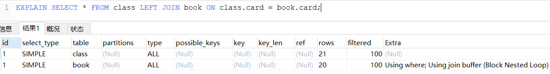• 分析：type 有 ALL ，rows 为表中数据总行数，说明 class 和 book 进行了全表检索

# 添加右表索引

• CREATE INDEX idx_book_card ON book(card);

• 分析语句：EXPLAIN SELECT * FROM class LEFT JOIN book ON class.card = book.card;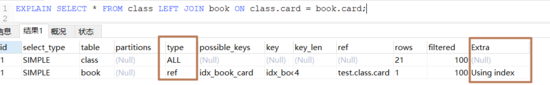• 分析：满足条件，故而删除当前索引，方便后续测试：DROP INDEX idx_book_card ON book;这是由左连接特性决定的。LEFT JOIN 条件用于确定如何从右表搜索行，左边一定都有，所以右边是我们的关键点，一定需要建立索引。左表连接右表 ，则需要拿着左表的数据去右表里面查，索引需要在 右表中建立索引

# 添加左表索引

• CREATE INDEX idx_class_card ON class(card);

• 分析语句：EXPLAIN SELECT * FROM class LEFT JOIN book ON class.card = book.card;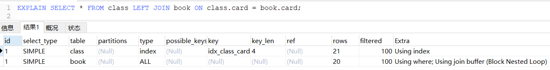• 分析：由上图可看出来索引未起到太大的作用，因为是左连接。左表驱动右边的时候由于 左表不论如何都需要循环遍历 ，故而 rows 还是原来的，其实这样并没有太大的效果

• 将左连接转成右连接 ：EXPLAIN SELECT * FROM class RIGHT JOIN book ON class.card = book.card;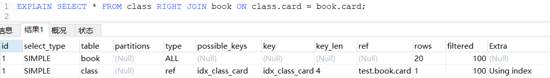• 分析：满足条件方便，后续测试删除当前索引：DROP INDEX idx_class_card ON class 这是因为 RIGHT JOIN 条件用于确定如何从左表搜索行，右边一定都有，所以左边是我们的关键点，一定需要建立索引。class RIGHT JOIN book ： book 里面的数据一定存在于结果集中 ，我们需要拿着 book 表中的数据，如 class 表中搜索，所以索引需要建立在 class 表中

# 建表

CREATE TABLE IF NOT EXISTS phone(    phoneid INT(10) UNSIGNED NOT NULL AUTO_INCREMENT,    card INT(10) UNSIGNED NOT NULL,    PRIMARY KEY(phoneid))ENGINE=INNODB;INSERT INTO phone(card) VALUES(FLOOR(1+(RAND()*20)));INSERT INTO phone(card) VALUES(FLOOR(1+(RAND()*20)));INSERT INTO phone(card) VALUES(FLOOR(1+(RAND()*20)));INSERT INTO phone(card) VALUES(FLOOR(1+(RAND()*20)));INSERT INTO phone(card) VALUES(FLOOR(1+(RAND()*20)));INSERT INTO phone(card) VALUES(FLOOR(1+(RAND()*20)));INSERT INTO phone(card) VALUES(FLOOR(1+(RAND()*20)));INSERT INTO phone(card) VALUES(FLOOR(1+(RAND()*20)));INSERT INTO phone(card) VALUES(FLOOR(1+(RAND()*20)));INSERT INTO phone(card) VALUES(FLOOR(1+(RAND()*20)));INSERT INTO phone(card) VALUES(FLOOR(1+(RAND()*20)));INSERT INTO phone(card) VALUES(FLOOR(1+(RAND()*20)));INSERT INTO phone(card) VALUES(FLOOR(1+(RAND()*20)));INSERT INTO phone(card) VALUES(FLOOR(1+(RAND()*20)));INSERT INTO phone(card) VALUES(FLOOR(1+(RAND()*20)));INSERT INTO phone(card) VALUES(FLOOR(1+(RAND()*20)));INSERT INTO phone(card) VALUES(FLOOR(1+(RAND()*20)));INSERT INTO phone(card) VALUES(FLOOR(1+(RAND()*20)));INSERT INTO phone(card) VALUES(FLOOR(1+(RAND()*20)));INSERT INTO phone(card) VALUES(FLOOR(1+(RAND()*20)));View Code

# 查询案例：

• 实现三表联查：SELECT * FROM class LEFT JOIN book ON class.card = book.card LEFT JOIN phone ON book.card = phone.card

• 分析语句：EXPLAIN SELECT * FROM class LEFT JOIN book ON class.card = book.card LEFT JOIN phone ON book.card = phone.card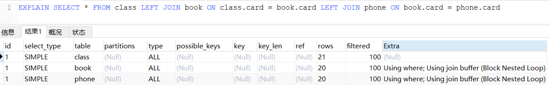• 结论：type 有 ALL，rows 为表数据总行数，说明 class、 book 和 phone 表都进行了 全表检索 Extra 中 Using join buffer ，表明连接过程中使用了 join 缓冲区

# 创建索引：

• ALTER TABLE book ADD INDEX x (card); ALTER TABLE phone ADD INDEX y (card);

• 分析语句：EXPLAIN SELECT * FROM class LEFT JOIN book ON class.card = book.card LEFT JOIN phone ON book.card = phone.card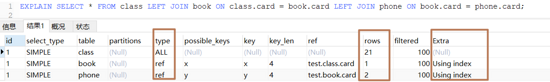• 分析：进行 LEFT JOIN ，永远都在右表的字段上建立索引

# 4，总结

1. 尽可能 减少 Join 语句中的 NestedLoop 的循环总次数 ；

2. 永远用 小结果集驱动大的结果集 （在 大结果集中建立索引 ，在小结果集中遍历全表）；

3. 优先优化 NestedLoop 的 内层循环 ；

4. 保证 Join 语句中被驱动表上 Join 条件字段已经被索引 ；

5. 当无法保证被驱动表的 Join 条件字段被索引且内存资源充足的前提下，不要太吝惜 JoinBuffer 的设置；

# 创建表：

CREATE TABLE staffs(    id INT PRIMARY KEY AUTO_INCREMENT,    name VARCHAR(24)NOT NULL DEFAULT'' COMMENT'姓名',    age INT NOT NULL DEFAULT 0 COMMENT'年龄',    pos VARCHAR(20) NOT NULL DEFAULT'' COMMENT'职位',    add_time TIMESTAMP NOT NULL DEFAULT CURRENT_TIMESTAMP COMMENT'入职时间')CHARSET utf8 COMMENT'员工记录表';INSERT INTO staffs(name,age,pos,add_time) VALUES('z3',22,'manager',NOW());INSERT INTO staffs(name,age,pos,add_time) VALUES('July',23,'dev',NOW());INSERT INTO staffs(name,age,pos,add_time) VALUES('2000',23,'dev',NOW());View Code

# 1，索引失效准则

1. 全值匹配我最爱

2. 最佳左前缀法则 ：如果索引了多例，要遵守最左前缀法则。指的是 查询从索引的最左前列开始并且不跳过索引中的列 。

3. 不在索引列上做任何操作 （计算、函数、（自动 or 手动）类型转换），会导致索引失效而转向全表扫描

4. 存储引擎 不能使用索引 中 范围条件右边的列

5. 尽量使用覆盖索引（只访问索引的查询（ 索引列和查询列一致 ））， 减少 select *

6. mysql 在使用 不等于 （!=或者<>）的时候无法使用索引会导致 全表扫描 (并不绝对，需考虑 成本 问题，例如 id!=''时还是会用到索引的)

7. is null ， is not null 也无法使用索引（并不绝对，需考虑 成本 问题）

8. like 以通配符开头（’%abc…’）mysql 索引失效会变成全表扫描操作(如果是'a%'则使用 range 索引)

9. 字符串不加引号索引失效

10. 少用 or ，用它连接时会索引失效

# a)创建复合索引

#创建复合索引ALTER TABLE staffs ADD INDEX index_staffs_nameAgePos(name,age,pos);#查看索引SHOW INDEX FROM staffs;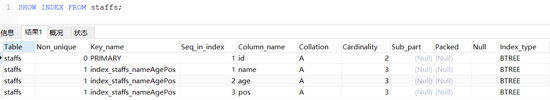# b)where 条件匹配

• 当顺序匹配时：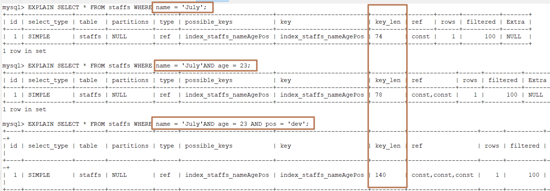• 当 不存在最左索引 （name）时：可以看到此时索引失效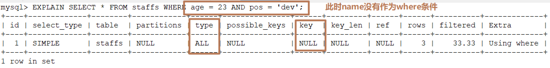• 当 中间索引不存在 （age）时：可以看到此时有效索引只有一个 const，即：只有 name 有效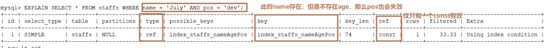• 当再 索引列上计算 或者使用函数时，会导致索引失效：使用 left(name,4)='July'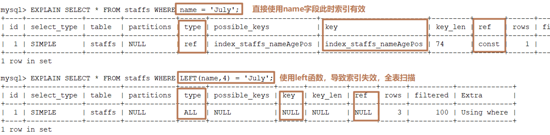• 当使用 范围索引 之后，后续的索引就会失效：name 条件不变，把 age 由原来的等于变成大于，此时索引类型就由 ref -> range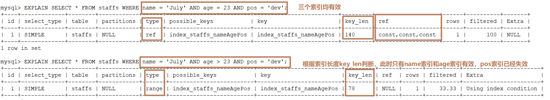• 尽量 使用覆盖索引 （只访问索引的查询（ 索引列和查询列一致 ）），减少 select *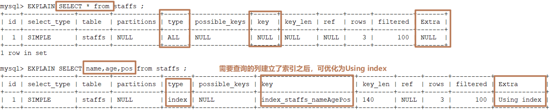• like 中使用 %的位置决定是否使用索引(如果 %在左边或者左右都有索引会失效，如果 %只在右边索引有效)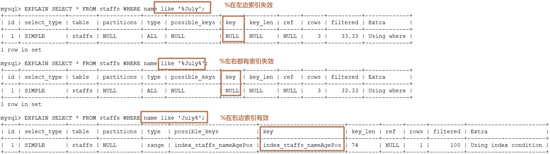• 如果确实是 需要将 %放在左边 可采用 覆盖索引优化 (只查询需要的列并命中到索引上)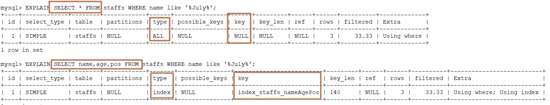• 字符串不加单引号索引失效 ：name=2000 和 name='2000'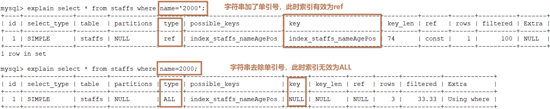• 少用 or ，用它连接时会索引失效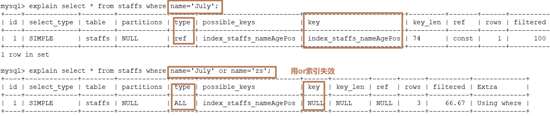• mysql 在使用 不等于 （!=或者<>）的时候无法使用索引会导致全表扫描(当然也并不绝对，这里有一个回执成本问题)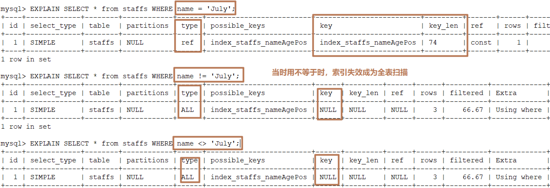• is null，is not null 会导致索引失效：key = null 表示索引失效(并不绝对，会考虑成本问题)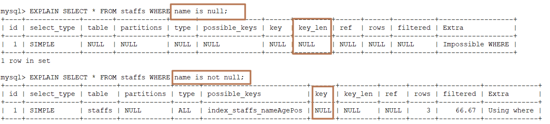成本。对于使用二级索引（innodb）进行查询来说，成本组成主要有两个方面：    读取二级索引记录的成本    将二级索引记录执行回表操作，也就是到聚簇索引中找到完整的用户记录的操作所付出的成本。很显然，要扫描的二级索引记录条数越多，那么需要执行的回表操作的次数也就越多，达到了某个比例时，使用二级索引执行查询的成本也就超过了全表扫描的成本（举一个极端的例子，比方说要扫描的全部的二级索引记录，那就要对每条记录执行一遍回表操作，自然不如直接扫描聚簇索引来的快）。所以MySQL优化器在真正执行查询之前，对于每个可能使用到的索引来说，都会预先计算一下需要扫描的二级索引记录的数量。所以对于以上三种查询条件是否会命中索引就取决于二级索引查询的成本与全局查询成本的高低。

# 1，建表

create table test03(    id int primary key not null auto_increment,    c1 char(10),    c2 char(10),    c3 char(10),    c4 char(10),    c5 char(10));insert into test03(c1,c2,c3,c4,c5) values ('a1','a2','a3','a4','a5');insert into test03(c1,c2,c3,c4,c5) values ('b1','b2','b3','b4','b5');insert into test03(c1,c2,c3,c4,c5) values ('c1','c2','c3','c4','c5');insert into test03(c1,c2,c3,c4,c5) values ('d1','d2','d3','d4','d5');insert into test03(c1,c2,c3,c4,c5) values ('e1','e2','e3','e4','e5');#创建复合索引create index idx_test03_c1234 on test03(c1,c2,c3,c4);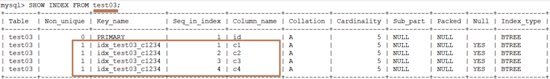# 2，案例

#只有whereEXPLAIN SELECT * FROM test03 WHERE c1='a1' AND c2='a2' AND c3='a3' AND c4='a4';EXPLAIN SELECT * FROM test03 WHERE c4='a4' AND c3='a3' AND c2='a2' AND c1='a1';EXPLAIN SELECT * FROM test03 WHERE c1='a1' AND c2='a2' AND c3>'a3' AND c4='a4';EXPLAIN SELECT * FROM test03 WHERE c1='a1' AND c2='a2' AND c4>'a4' AND c3='a3';#where条件与order by 结合EXPLAIN SELECT * FROM test03 WHERE c1='a1' AND c2='a2' AND c4='a4' ORDER BY c3;EXPLAIN SELECT * FROM test03 WHERE c1='a1' AND c2='a2' ORDER BY c3;EXPLAIN SELECT * FROM test03 WHERE c1='a1' AND c2='a2' ORDER BY c4;EXPLAIN SELECT * FROM test03 WHERE c1='a1' AND c5='a5' ORDER BY c2, c3;EXPLAIN SELECT * FROM test03 WHERE c1='a1' AND c5='a5' ORDER BY c3, c2;EXPLAIN SELECT * FROM test03 WHERE c1='a1' AND c2='a2' ORDER BY c2, c3;EXPLAIN SELECT * FROM test03 WHERE c1='a1' AND c2='a2' AND c5='a5' ORDER BY c2, c3;EXPLAIN SELECT * FROM test03 WHERE c1='a1' AND c2='a2' AND c5='a5' ORDER BY c3, c2;#where与group by结合EXPLAIN SELECT * FROM test03 WHERE c1='a1' AND c4='a4' GROUP BY c2, c3;EXPLAIN SELECT * FROM test03 WHERE c1='a1' AND c4='a4' GROUP BY c3, c2;

# a）where 查询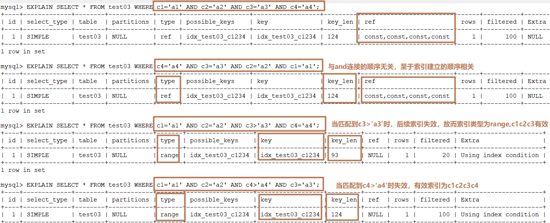# b）where 与 order by 结合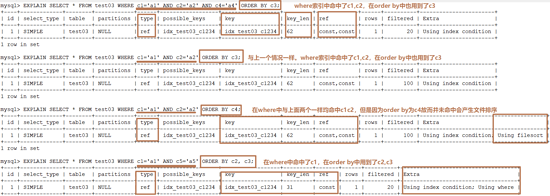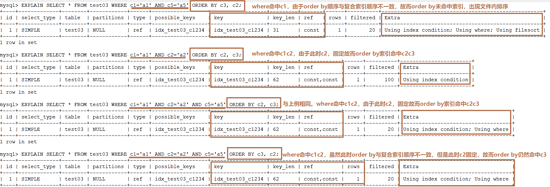# c）where 与 group by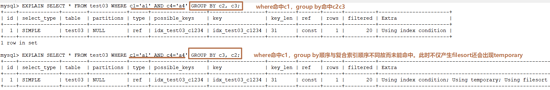# a)建议

1. 对于单键索引，尽量选择针对当前 query 过滤性更好的索引

2. 在选择 组合索引 的时候，当前 query 中 过滤性最好的字段 在索引字段顺序中，位置 越靠左越好 。

3. 在选择 组合索引 的时候，尽量选择可以能包含当前 query 查询条件中 where 子句更多字段的索引

4. 尽可能通过分析统计信息和调整 query 的写法来达到选择合适索引的目的

# b)案例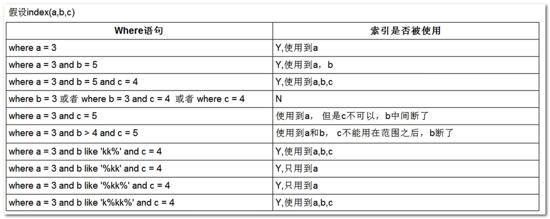### 程序员小毕

Java领域;架构知识;面试心得;互联网行业最新资讯

## 评论